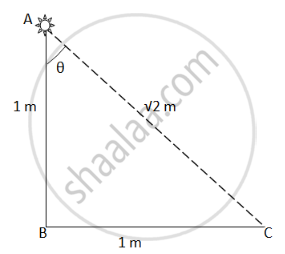Department of Pre-University Education, KarnatakaPUC Karnataka Science Class 12

# An Electric Bulb is Hanging Over a Table at a Height of 1 M Above It. the Illuminance on the Table Directly Below the Bulb is 40 Lux - Physics

MCQ
Fill in the Blanks

An electric bulb is hanging over a table at a height of 1 m above it. The illuminance on the table directly below the bulb is 40 lux. The illuminance at a point on the table 1 m away from the first point will be about ___________ .

• 10 lux

• 14 lux

• 20 lux

• 28 lux

#### Solution

14 luxHere,

$r = \sqrt{2}$

$\tan\theta = \frac{BC}{AB} = 1$

$I_o = 40\text{ lux}$

$\theta = \tan^{- 1} \left( 1 \right) = {45}^0$

The illuminance is given by

$E = \frac{I_o \cos\theta}{r^2}$

$= \frac{40 \times \cos( {45}^0 )}{\left( \sqrt{2} \right)^2}$

$= 14\text{ lux}$

Concept: Light Process and Photometry
Is there an error in this question or solution?

#### APPEARS IN

HC Verma Class 11, Class 12 Concepts of Physics Vol. 1
Chapter 22 Photometry
MCQ | Q 4 | Page 454
Share